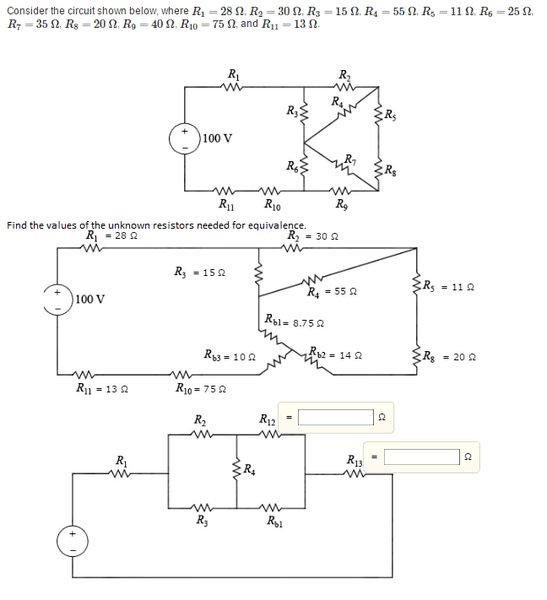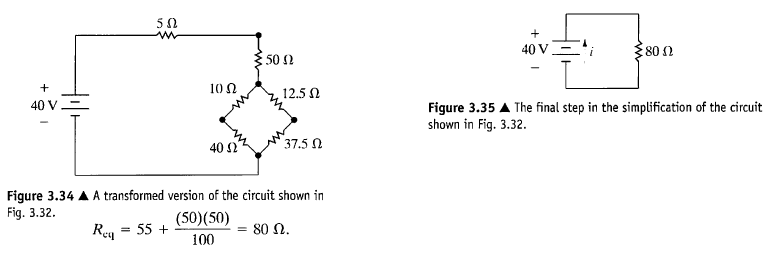# Delta-Wye Circuit

• Engineering

## Homework Statement

R1=28Ω, R2=30Ω, R3=15Ω, R4=55Ω, R5=11Ω, R6=25Ω, R7=35Ω, R8=20Ω, R9=40Ω, R10=75Ω, and R11=13Ω.
Determine the current through resistor R3.
Find the values of the unknown resistors needed for equivalence.
http://‪C:\Users\me\Desktop\deltawye.png http://‪C:\Users\me\Desktop\deltawye.png http://‪C:\Users\me\Desktop\deltawye.png

## Homework Equations

Rab = Rc(Ra + Rb)/ (Ra+Rb+Rc) = R1 + R2

## The Attempt at a Solution

I transformed the bottom delta in the first circuit in the attached image into a wye using the above equation to get the second circuit. However, I'm having trouble finding the values of R12 and R13 in the third image. I'm not sure how to tell what resistors are in series or in parallel with each other in the second circuit once a transformation is done. I thought that in the second circuit that R11 , R10 , Rb2 , Rb3 , R8 , and R5 were all in series with each other, so I added up the values of those resistors to get 143 ohms as the value of R13 in the third circuit. However, I don't understand then, what the value of R12 could be in that case. I'm also not sure how to tell what resistors are in series or in parallel when the transformed circuit looks like the second attached image. In that circuit, I had thought that the 10, 12.5, 40, and 37.5 ohm resistors were in series with each other, and that those were also in series with the 50 and 5 ohm resistors, but I know that doesn't make any sense. How would I be able to find the equivalent resistance in the circuits in those two images?

I would really appreciate any help on this. Thanks so much.The Electrician
Gold Member
R12 is R5+R8+Rb2. R13 is R11+R10+Rb3.

Thanks so much for your help! So, if R12 = 45 and R13 = 98, and then I do another delta to wye transformation like in the image attached, how could I use this information to solve for the current I3? I think the hint given in the problem says that we wanted to reduce the first circuit until R3 is part of a parallel branch. How would that help us exactly? I know that the voltage across resistors in parallel is the same, so could I use that to help me figure out the current through I3? Or, could I maybe use current division to solve this?

Also, in the attached image, is the equivalent resistance 143.818 ohms? Because wouldn't R2 and R14 be in series with other and R3 and R15 would be in series with other, but R2+R14 would be in parallel with R3+ R15, and then that equivalent resistance would be in series with R1, R16, and R13?

#### Attachments

Last edited:
The Electrician
Gold Member
R2 and R14 can be replaced with an equivalent single resistor; call it R17. R3 and R15 can be replaced with an equivalent single resistor; call it R18.

R17 and R18 are in parallel and can be replaced with an equivalent single resistor R19.

R1, R19, R16 and R13 are in series across the 100V supply. You can calculate the current through that series circuit. That same current will flow through R19. You can then calculate the voltage across R19; the voltage across R19 is the same as the voltage across the series combination of R3 and R15. Knowing the resistance of R3+R15 and the voltage across that series combination, we can calculate the current through R3 and R15. The current through R3 is what you're supposed to find. Post your result.

•abcxyz123
Hi, thanks so much for your response. So, the equivalent resistance for the whole circuit is 143.82 ohms, the current through that series circuit is .6953 amps which would also be the current through R19. So, since R19 is 14.198 ohms, the voltage across R19 is 9.87 V which is the same as the voltage across R18. Since R18 = R3 + R15 = 19.42 ohms, the current through R18 would be .508 amps and since R3 and R15 are in series, they have the same current as R18, so the current through R3 would be .508 amps. Is that the correct way to think about it?

Also, in the second image in my first post, figure 3.34, how would I find the equivalent resistance of that circuit? Are the 10 and 40 ohms in series with each other and then 12.5 and 37.5 ohms are in series with each other, and then those would be in parallel with each other and that whole diamond shape would then be in series with the 5 and 50 ohms?

Last edited:
The Electrician
Gold Member
Also, in the second image in my first post, figure 3.34, how would I find the equivalent resistance of that circuit? Are the 10 and 40 ohms in series with each other and then 12.5 and 37.5 ohms are in series with each other, and then those would be in parallel with each other and that whole diamond shape would then be in series with the 5 and 50 ohms?

Exactly right.

Thanks so much for your help! :)